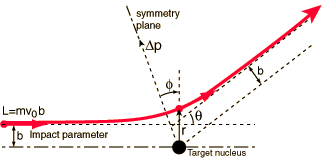# How does the kinetic energy of the alpha particles affect the angle of deflection?

Jul 16, 2014

Alpha particles with less kinetic energy are deflected through greater angles than more energetic ones.When you calculate the scattering angle θ as a function of the kinetic energy $E$ of the α particle, you get the equation

(2bE)/(Z_1Z_2e^2) = cot(θ/2), where

$b$ is the impact parameter — the distance of closest approach if the α particle were not deflected
${Z}_{1}$ and ${Z}_{2}$ are the charges on the α particle and the gold nucleus
$e$ is the electronic charge

For a fixed value of $b$, the equation says that

E ∝ cot(θ/2) or

θ ∝ arccot($E$)

A plot of $y$ =arccot($x$) or θ = arccot($E$) looks like this:Since $E$ is always positive, we need to look at only the right hand side of the plot.

We see that as the kinetic energy $E$ increases, the deflection angle θ decreases. Also, as the kinetic energy $E$ decreases, the deflection angle θ increases.